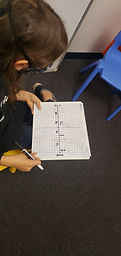Mr. Jake

Target 1​

Lesson Type:

New

Data Probability

:

Interpreting Data

Construct and read graphs from given information to solve problems.

1:

Construct and read simple line plots depicting measurement data.

6th

Vocabulary:

Line Plot, Data, Range

Activities:

Collected data and used the information to create a line plotHome Exploration

Guiding Questions:Absent Students:

Target 2

:

1:

Accurately measure angles.

2:

Understand that angles are measured in degrees (from 0 to 360).

3:

Understand that angles can be composed or decomposed, using addition and subtraction.

4:

Understand that an angle is the union of two rays with a common endpoint (vertex).

6th

Vocabulary:

Protractor, Angle, Measurement

Activities:

Used protractor to measure angles.Home Exploration

Guiding Questions:Target 3

:

Vocabulary:

Activities:Home Exploration